Sequential Circuits

Sequential circuits are those with memory, also called “feedback”.  In this, they differ
from combinational circuits, which have no memory.

The stable output of a combinational circuit does not depend on the order in which its
inputs are changed.  The stable output of a sequential circuit usually does depend
on the order in which the inputs are changed.

We usually focus on clocked sequential circuits, in which the circuit changes state
at fixed times in a clock cycle.  Clocked circuits are easier to design and understand.

All sequential circuits depend on a phenomenon called gate delay.  This reflects the fact
that the output of any logic gate (implementing a Boolean function) does not change
immediately when the input changes, but only some time later.

The gate delay for modern circuits is typically a few nanoseconds.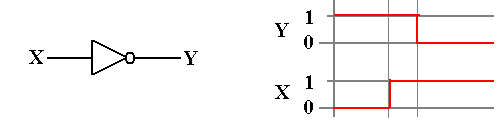Another Circuit Dependent on Gate Delay

The following is a statement of the Inverse Law of Boolean Algebra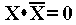But consider the following circuit and its timing diagram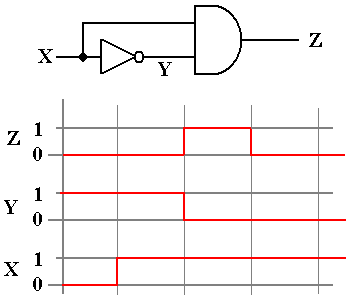Note that for a short time (one gate delay) we have Z = 1.

This is a pulse generator.

A NOR Gate with Feedback

We consider yet another circuit that is based on gate delays.

This is based on the NOR gate, which is an OR gate followed by a NOT gate.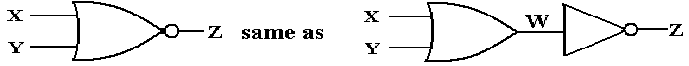Now consider the truth table for the NOR gate, which we build from the truth tables for the OR gate and the NOT gate.

 X Y W Z 0 0 0 1 0 1 1 0 1 0 1 0 1 1 1 0

The important thing to note is can be expressed in two equations.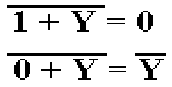A NOR Gate with Feedback (Part 2)

Consider the following circuit.  When X = 1, Z = 0, and Y = 0.  It is stable.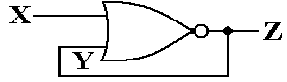The behavior becomes interesting when X = 0.  Just after X becomes 0, we still
have Z = 0 and Y = 0 due to gate delays.  But NOR(0, 0) = 1, so after one gate delay,
we have Z = 1 and Y = 1.  But NOR(0, 1) = 0, so after another gate delay, we have Z = 0.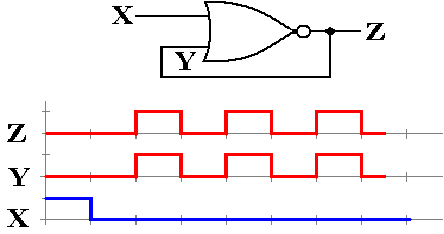This might be used to generate a system clock, though it probably lacks the stability and accuracy that are normally expected of such a device.

Cross–Coupled NOR Gates: The SR Latch

Consider the following circuit.  Each of the two NOR gates has two inputs, one from an external source and one that is fed back from the other NOR gate.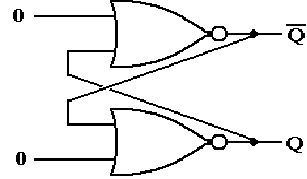Here the two external inputs are 0 and 0.  We do not specify the outputs, except to require that one is the complement of the other; if Q = 1, then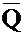= 0, and vice versa.

The two inputs to the top NOR gate are 0 and Q.  But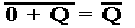so this part of the circuit is stable.

The two inputs to the bottom NOR gate are 0 and.  But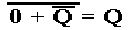so this part of the circuit is also stable.

The circuit is stable with external inputs of 0 and 0.

The SR Latch (Part 2)

Let the above be in either state (Q = 0 or Q = 1) and change the external input.
At first, the output does not change (remember the gate delays).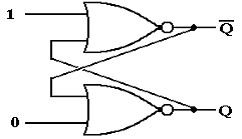After one gate delay, the output of the top NOR gate changes to 0.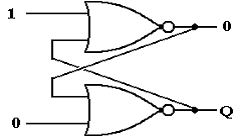The inputs to the bottom NOR gate are now 0 and 0.  After another gate delay it changes.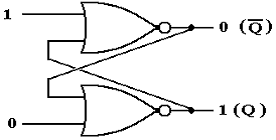The SR Latch (Part 3)

Let the above be in either state (Q = 0 or Q = 1) and change the external input.
At first, the output does not change (remember the gate delays).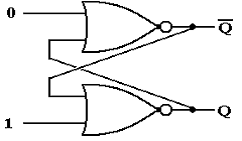After one gate delay, the output of the bottom NOR gate changes to 0.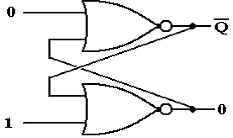The inputs to the top NOR gate are now 0 and 0; its output changes to 1.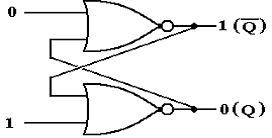The SR Latch (Part 4)

We now have a basic memory device, which stores one bit denoted as Q.
Here is the general circuit diagram of the device.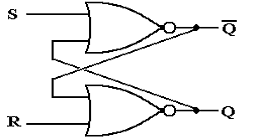So, we have a device that can store a single bit, with the following options:

1.     Store a 0

2.     Store a 1

3.     Retain the current contents.

The behavior of such a memory device is described by its characteristic table.

The SR Latch (Part 5)

What about the other input: S = 1 and R = 1.

Remember that if one input of a NOR gate is logic 1, its output is logic 0.
Specifically for any value of Q, we have: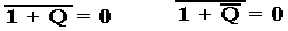This leads to the circuit with the following stable state.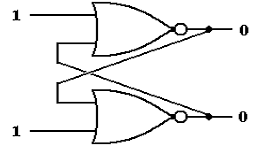But we cannot have both Q = 0 and= 0.

At this point, we have two options:
1.     Give up on using this device as a memory device, or
2.     Disallow the inputs S = 1 and R = 1.  We choose this option.
S = 1 and R = 1 will be labeled as the next state to be an ERROR.

The SR Characteristic Tables

Here is the complete version of the characteristic table.  It has eight rows.

 S R Present State Next State 0 0 0 0 0 0 1 1 0 1 0 0 0 1 1 0 1 0 0 1 1 0 1 1 1 1 0 ERROR 1 1 1 ERROR

We almost always abbreviate the above table as follows.

 S R Next State 0 0 Present State 0 1 0 1 0 1 1 1 ERROR

The SR Excitation Tables

Excitation tables present a slightly different view of flip–flops.
These tables are logically equivalent to the characteristic tables, but presented differently.

If the Present State is 0 and the desired Next State is 0, we have two options:
S = 0 and R = 0       This keeps the state the same.
S = 0 and R = 1       This forces the state to 0.

This is abbreviated as S = 0 and R = d (don’t care), because if the Present State is 0 and
S = 0, the Next State will be 0 without regard to the value of R.

If the Present State is 0 and the desired Next State is 1, we must have S = 1 and R = 0.

If the Present State is 1 and the desired Next State is 0, we must have S = 0 and R = 1.

If the Present State is 1 and the desired Next State is 1, we again have two options:
S = 0 and R = 0       This keeps the state the same.
S = 1 and R = 0       This forces the state to 1.       Thus, S = d and R = 0.

Here is the Excitation Table for an SR Latch.

 PS NS S R 0 0 0 d 0 1 1 0 1 0 0 1 1 1 d 0

Clocked Latches and Flip–Flops

Both latches and flip–flops store a single binary value, often called the “state”.

Latches of the sort just discussed can lead to unpredictable instabilities.  Our goal will be to have a circuit element with a definite present state, rules for generating its next state, and some control over when the device changes state.

The system clock provides a mechanism by which we may have circuits that meet this design criterion.  These are called “synchronous circuits”.

Both clocked latches and flip–flops change state only at fixed intervals of a repeating signal called the system clock.  We now denote the states as follows.

The present state of the flip–flop is often called Q(t)
The next state of the flip–flop is often called Q(
t + 1)

The sequence: the present state is Q(t), the clock “ticks”, the state is now Q(t + 1)

Denote the present time by the symbol t.  Since our focus is only on how a circuit element transitions from one state to the next, we do not worry about actual clock time.

In other words, the next time period is called t + 1, even if it is 0.5 nanoseconds later.

Views of the System Clock

There are a number of ways to view the system clock.  In general, the view depends on the detail that we need in discussing the problem.  The logical view is shown in the next figure, which illustrates some of the terms commonly used for a clock.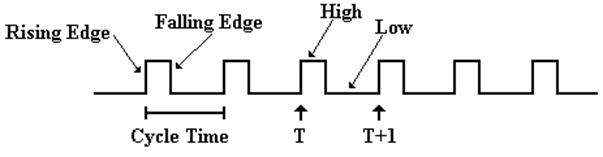The cycle time will be denoted by the Greek letter t.

The clock is typical of a periodic function.  There is a period t for which

f(t) = f(t + t)

This clock is asymmetric.  It is often the case that the clock is symmetric, where the time spent at the high level is the same as that at the low level.  Your instructor often draws the clock as asymmetric, just to show that such a design is allowed.

Clock Period and Frequency

If the clock period is denoted by t, then the frequency (by definition) is f = 1 / t.

For example, if t = 2.0 nanoseconds, also written as t = 2.0·10–9 seconds, then
f = 1 /
(2.0·10–9 seconds) = 0.50·109 seconds–1 or 500 megahertz.

If f = 2.5 Gigahertz, also written as 2.5·109 seconds–1, then
t = 1.0 / (2.5·109 seconds–1) = 0.4·10–9 seconds = 0.4 nanosecond.

Views of the System Clock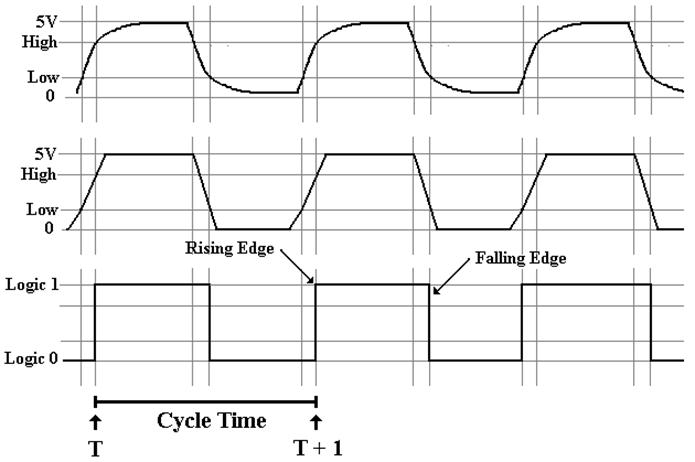The top view is the “real physical view”.  It is seldom used.
The middle view reflects the fact that voltage levels do not change instantaneously.

The Clocked SR Latch

Clocked latches are sometimes called “level triggered flip–flops”.  These are sensitive to their input only during one phase of the clock, either when it is high or it is low.

We describe a clocked latch that is sensitive to its input only when the clock is high.
Here is the requirement in terms of the clock.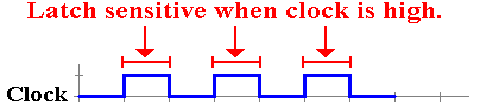Here is the circuit that implements this design criterion.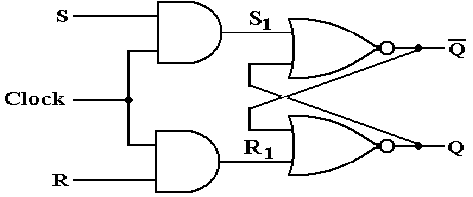Note that when Clock = 0, we have S1 = 0 and R1 = 0 without regard to the values of
S and R.  The latch does not change.  When Clock = 1, we have S1 = S and R1 = R.

The Clocked D Latch

We now introduce a variant of the SR latch that is specialized to store data.

It is the D Latch, also called the “Data Latch”.  It will store data on every clock pulse.When Clock = 0,                    then each of S1 and R1 is 0.    No change in state.

When Clock = 1 and D = 0,   then S1 = 0 and R1 = 1.           Latch is cleared; Q = 0.

When Clock = 1 and D = 1,   then S1 = 1 and R1 = 0.          Latch is set; Q = 1.

The D Latch and the D Flip–flop (to be defined soon) are quite useful in building devices that store data.  Examples are registers and I/O interface controls.

The Feedback Problem

Clocked latches have one major problem: they are sensitive to input for too long a time.

Overly extended sensitivity to input can lead to instabilities.

Consider the following problem, which is routinely addressed in a computer.

There is a register called the PC (Program Counter).
As a regular part of program execution it is incremented: PC
¬ (PC) + 1.

This operation is so frequently done that every CPU has a dedicated path for it.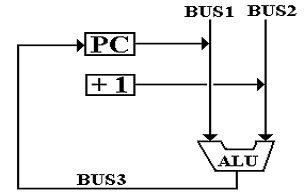There is a dedicated constant register permanently set to hold the value 1.

The ALU adds 1 to the contents of the PC and feeds the result back to the PC.

The Feedback Problem (Part 2)

Suppose the Program Counter is implemented as a set of D Latches.

Problems will occur if the feedback comes at the wrong phase of the clock.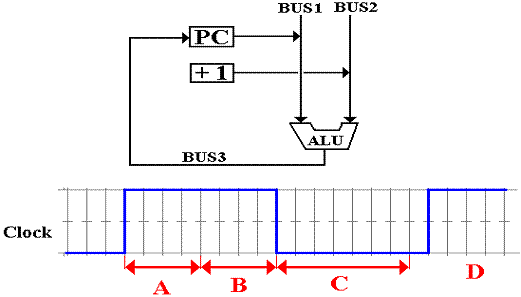The addition operation begins just after the rising edge of the clock.  If the result of the addition reaches the set of latches holding the PC too soon, we have problems.
A     This will definitely cause problems.  The PC will be incremented by 2.
B     This might cause problems.
C     This is the best time for the feedback to arrive at the latches.
D     The feedback is too late; the PC is not incremented.  This rarely occurs.

The Feedback Problem (Part 3)

We need to reduce the time during which the register will accept input.

What we need is reflected in the following timing diagram.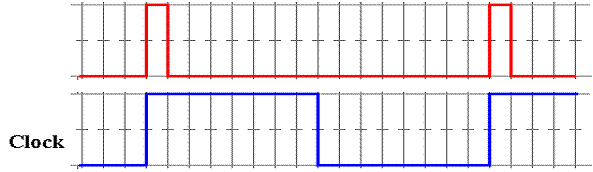Suppose a circuit that causes the latches to be sensitive to input for only one gate delay.

The ALU will have at least one gate in its circuitry, as do the modified latches for the PC.

As a result, the feedback cannot get back to the PC while it is still sensitive to input.
Instabilities cannot occur.

We can modify the latch by adding a pulse generator, discussed previously.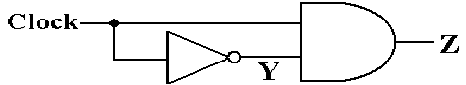A latch so modified is called a Flip–Flop.

The SR and D Flip–Flops

Here is the circuit diagram for an SR flip–flop that is fabricated from NOR gates.
One can also fabricate it from NAND gates, but we ignore this option.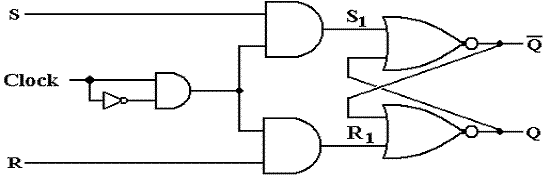Here is the circuit diagram for a D flip–flop that is fabricated from NOR gates.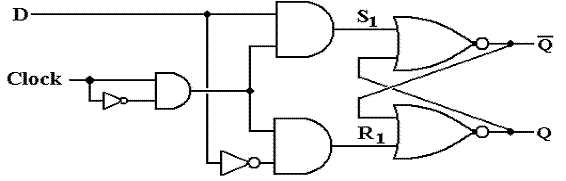We Need Another Flip–Flop

Consider the characteristic table for the SR flip–flop.

It is the same as that for the SR latch, except for the explicit reference to the clock.

 S R Q(t + 1) 0 0 Q(t) 0 1 0 1 0 1 1 1 ERROR

Were we to modify the SR flip–flop, what could be placed in the last row?

It is easy to see that there are only four Boolean functions of a single Boolean variable Q.
F(Q) = 0, F(Q) = Q, F(Q) =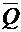, and F(Q) = 1.  The above table is missing.

This gives rise to the JK, the most general of the flip–flops.  Its characteristic table is:

 J K Q(t + 1) 0 0 Q(t) 0 1 0 1 0 1 1 1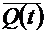The JK Flip–Flop

One can modify an SR flip–flop to have the desired characteristics.

It can be shown that the following circuit does the job.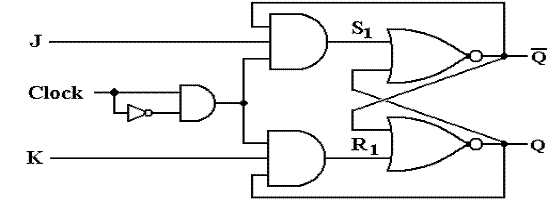It can be shown that the circuit above is logically equivalent to the next one.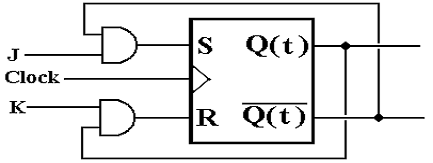The second circuit slightly facilitates the proof that the JK operates as advertised.

Design and Analysis Based on Flip–Flops

We have shown the detailed construction of flip–flops only to show that they work.

We now move to issues associated with the use of flip–flops.

For these issues, we begin to view the devices as “bit boxes” that somehow store the binary data with the proper timings.

We focus on two tables for each flip–flop.

The characteristic table specifies the next state for the present state and input.

The excitation table specifies the input required to take the flip–flop from the
given present state to the desired next state.

 Table Type Given This Determine This Characteristic Present State and Input Next State Excitation Present State and Desired Next State Input required.

We now proceed with this view of the flip–flop.

SR Flip–Flop

Here is the diagram for the SR flip–flop.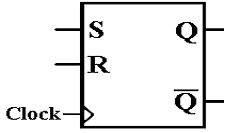Here again is the state table for the SR flip–flop.

 S R Q(t + 1) 0 0 Q(t) 0 1 0 1 0 1 1 1 Error

Here is the Excitation Table for an SR Flip–Flop.

 Q(t) Q(t + 1) S R 0 0 0 d 0 1 1 0 1 0 0 1 1 1 d 0

JK Flip–Flop

A JK flip–flop generalizes the SR to allow for both inputs to be 1.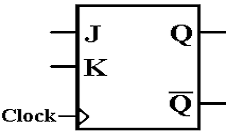Here is the characteristic table for a JK flip–flop.

 J K Q(t + 1) 0 0 Q(t) 0 1 0 1 0 1 1 1Here is the Excitation Table for a JK Flip–Flop.

 Q(t) Q(t + 1) J K 0 0 0 d 0 1 1 d 1 0 d 1 1 1 d 0

The D Flip–Flop

The D flip–flop specializes either the SR or JK to store a single bit.  It is very useful for interfacing the CPU to external devices, where the CPU sends a brief pulse to set the value in the device and it remains set until the next CPU signal.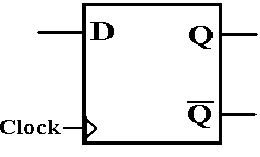The characteristic table for the D flip–flop is so simple that it is expressed better as the equation Q(t + 1) = D.  Here is the table.

 D Q(t + 1) 0 0 1 1

The excitation table for a D flip–flop is usually not given.
It is better represented by an equation D = Q(
t + 1).

The T Flip–Flop

The “toggle” flip–flop allows one to change the value stored.  It is often used in circuits in which the value of the bit changes between 0 and 1, as in a modulo–4 counter in which the low–order bit goes 0, 1, 0, 1, 0, 1, etc.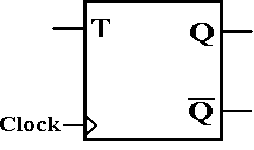The characteristic table for the T flip–flop is so simple that it is expressed better as the equation Q(t + 1) = Q(t) Å T.  Here is the table.

 T Q(t + 1) 0 Q(t) 1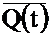We could give an excitation table for the T flip–flop, but the idea is better expressed by the excitation equation.

T = Q(t) Å Q(t + 1)

The JK Flip–Flop as a General–Use Flip–Flop

The JK flip–flop can be used to implement any of the other three flip–flops.

As a D flip–flop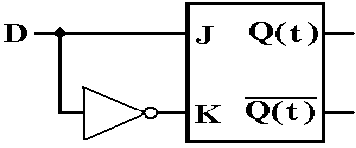As a T flip–flop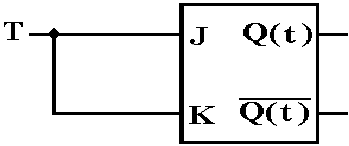As an SR flip–flop.  Just never use J = 1 and K = 1 as simultaneous inputs.

A Real JK Flip–Flop

All real flip–flops have additional inputs.  Here is a more complete diagram.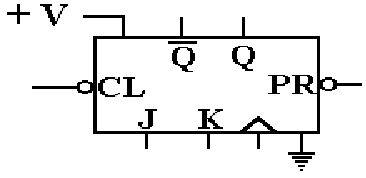First the flip–flop has a voltage input (usually 5 volts) and a ground attachment.
All flip–flops are electrical devices that require power and ground.

The flip–flop has a clock input that enables it to change state.  The triangle
indicates that this is an edge–triggered device, a true flip–flop.

As an edge–triggered device, the flip–flop changes on the rising edge of the clock.
All flip–flops have asynchronous inputs that can take effect at any time.
Note the circles on these two inputs; they are active low.

CL   Clear       When this is set to logic zero, the flip–flop is cleared; Q = 0.

PR   Preset      When this is set to logic zero, the flip–flop is set to logic 1; Q = 1.

A Real JK Flip–Flop: The 74LS109A

Here is the circuit diagram of the 74LS109A, a dual JK flip–flop.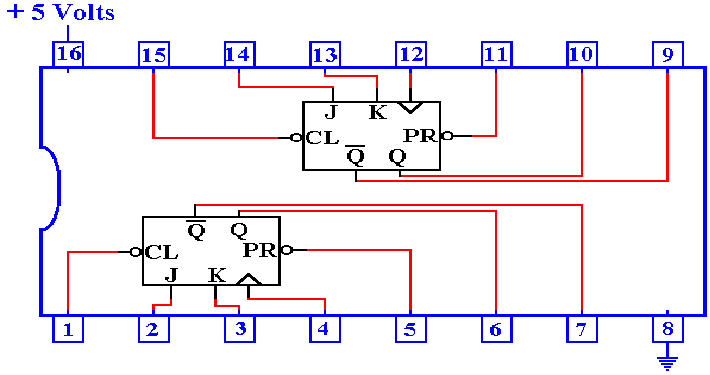Tri–State to Attach Flip–Flops to a Bus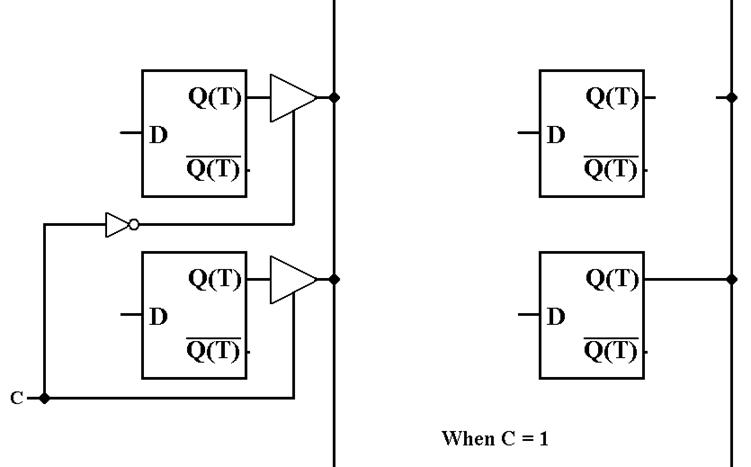The control unit must insure that at most one flip–flop is connected to the bus line.